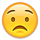# Problem using QImages (failing to save)

edited May 2020

Hello, I am trying to get the image of each shape in the layout for a specific layer. I could actually achieve that by using

``````lv.zoom_box(pya.DBox((l/dbu),(b/dbu), (r/dbu),(t/dbu)))
image_path = os.path.join(image_location, file_name + ".png")
lv.save_image(image_path,image_width,image_height)
``````

However, I guess, I need to use Qimage because later I want to add the center coordinates of each shape on the image as a text.
I try the following but it fails to successfully write the image``````my_q_image=lv.get_image_with_options (640, 480,1,0,0, pya.DBox(l/dbu, b/dbu, r/dbu, t/dbu),1)
check=my_q_image.save(image_path,'0',-1) #returns false if fail to write the image
print(check) #it is false :(
``````

Here is my full code. Thanks in advance! (Note: If you could also provide the code for writing text, I would really appreciate!)

``````    import pya
import os
mylayout = pya.Layout()

path=r'C:\Users\ACER\Desktop\Images\Cell4.oas'
image_location=r'C:\Users\ACER\Desktop\Images'
image_width=480
image_height=640

dbu=1000

app = pya.Application.instance()
mw = app.main_window()
mw.load_layout(path,0)  #load the layout to the mainwindow so that you can see it
lv = mw.current_view()

mycell=mylayout.cell(0) # is a pointer to the 'cell' object  (You can also refer to cell object with its name like: a=mylayout.cell(‘TOP’)
shapes = mycell.shapes(0)   #iterate through all shapes in a given layer for a given cell

counter=0
for i in shapes.each():
#print(i.box_center)  #center coordinates of of each box shape
#print(i.box) #bottom x , bottom y, upper x, upper y coordinates of the shape
l=i.box.left
b=i.box.bottom
r=i.box.right
t=i.box.top
counter=counter+1
file_name='shape'+str(counter)  #makes file name as shape1, shape2 ...
image_path = os.path.join(image_location, file_name + ".png")

#lv.zoom_box(pya.DBox((l/dbu),(b/dbu), (r/dbu),(t/dbu)))  #If you uncomment the following three lines it will work but then how to add a text?
#image_path = os.path.join(image_location, file_name + ".png")
#lv.save_image(image_path,image_width,image_height)

my_q_image=lv.get_image_with_options (640, 480,1,0,0, pya.DBox(l/dbu, b/dbu, r/dbu, t/dbu),1)
check=my_q_image.save(image_path,'0',-1) #returns false if fail to write the image
print(check) #it is false :(
``````

• Hi, Ege!

I tried next code on 0.26.4 on Linux and it worked for me:

```import pya

image = \
pya.Application.instance().main_window().current_view().get_image(640, 480)
print image.save("image_file.png")
```
• Thanks for the answer eugene!

• The second parameter to "QImage#save" should either be omitted or set to an empty string. 0 is a misleading because in C++ it's a "(const char *)0". There is no such thing in Python, but an empty string serves the same purpose.

Another symptom of automatic translation of the API.

Matthias